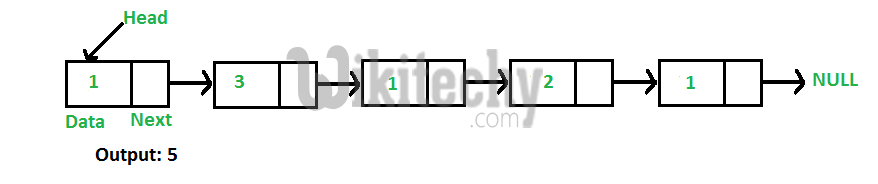# C Algorithm – Find Length of a Linked List both Iterative and Recursive

C Algorithm - Find Length of a Linked List both Iterative and Recursive - Linked List - Write a C function to count number of nodes in a given singly

Write a C function to count number of nodes in a given singly linked list.For example, the function should return 5 for linked list 1->3->1->2->1.

Iterative Solution

```1) Initialize count as 0
2) Initialize a node pointer, current = head.
3) Do following while current is not NULL
a) current = current -> next
b) count++;
4) Return count```

C Programming:

``````// Iterative C program to find length or count of nodes in a linked list
#include<stdio.h>
#include<stdlib.h>

struct node
{
int data;
struct node* next;
};

/* Given a reference (pointer to pointer) to the head
of a list and an int, push a new node on the front
of the list. */
void push(struct node** head_ref, int new_data)
{
/* allocate node */
struct node* new_node =
(struct node*) malloc(sizeof(struct node));

/* put in the data  */
new_node->data  = new_data;

/* link the old list off the new node */

/* move the head to point to the new node */
}

/* Counts no. of nodes in linked list */
{
int count = 0;  // Initialize count
struct node* current = head;  // Initialize current
while (current != NULL)
{
count++;
current = current->next;
}
return count;
}

/* Drier program to test count function*/
int main()
{

/* Use push() to construct below list
1->2->1->3->1  */

/* Check the count function */
printf("count of nodes is %d", getCount(head));
return 0;
}``````

Output:

`count of nodes is 5`

Recursive Solution

```int getCount(head)
1) If head is NULL, return 0.
2) Else return 1 + getCount(head->next)```

C Programming:

``````// Recursive C program to find length or count of nodes in a linked list
#include<stdio.h>
#include<stdlib.h>

struct node
{
int data;
struct node* next;
};

/* Given a reference (pointer to pointer) to the head
of a list and an int, push a new node on the front
of the list. */
void push(struct node** head_ref, int new_data)
{
/* allocate node */
struct node* new_node =
(struct node*) malloc(sizeof(struct node));

/* put in the data  */
new_node->data  = new_data;

/* link the old list off the new node */

/* move the head to point to the new node */
}

/* Counts the no. of occurences of a node
{
// Base case
return 0;

// count is 1 + count of remaining list
}

/* Drier program to test count function*/
int main()
{

/* Use push() to construct below list
1->2->1->3->1  */

/* Check the count function */
printf("count of nodes is %d", getCount(head));
return 0;
}``````

Output:

`count of nodes is 5`
READ  Cpp Algorithm-Point arbit pointer to greatest value right side node in a linked list#### Venkatesan Prabu

Wikitechy Founder, Author, International Speaker, and Job Consultant. My role as the CEO of Wikitechy, I help businesses build their next generation digital platforms and help with their product innovation and growth strategy. I'm a frequent speaker at tech conferences and events.